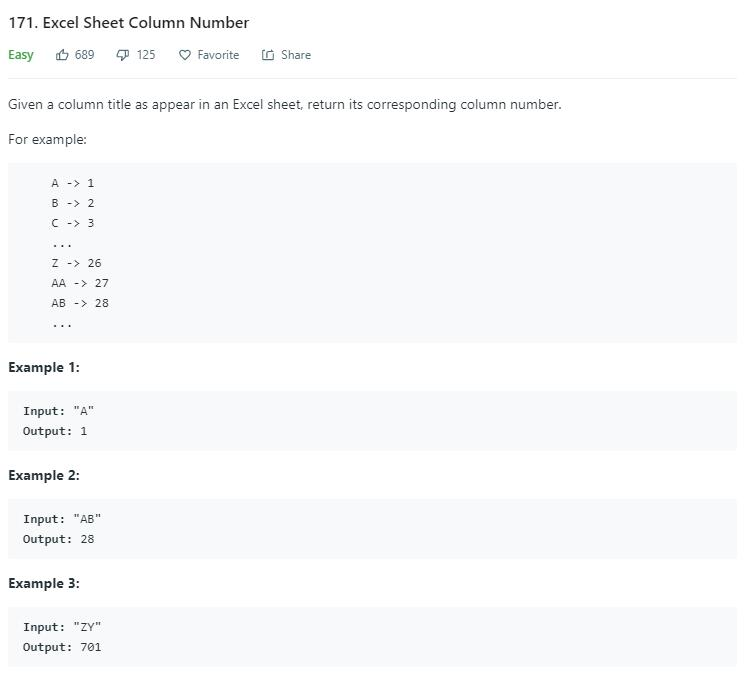# 题目描述（简单难度）# 解法一

$2\times10^3+0\times10^2+1\times10^1+9\times10^0=2019$

$...x_4\times26^3+x_3\times26^2+x_2\times26^1+x_1\times26^0$

public int titleToNumber(String s) {
char[] c = s.toCharArray();
int res = 0;
int mul = 1;
for (int i = c.length - 1; i >= 0; i--) {
res = res + mul * (c[i] - 'A' + 1);
mul *= 26;
}
return res;
}


c[i] - 'A' + 1 这里字符做差，就相当于 ASCII 码对应的数字做差，从而算出当前字母对应的数字。

# 解法二

int res = 0;
res = res * 10 + 2; //2
res = res * 10 + 0; //20
res = res * 10 + 1; //201
res = res * 10 + 9; //2019


$2\times10^3+0\times10^2+1\times10^1+9\times10^0=2019$

$10\times(2\times10^2+0\times10^1+1\times10^0)+9=2019$

$10\times(10\times(2\times10^1+0\times10^0)+1)+9=2019$

$10\times(10\times(10\times(10\times0 + 2)+0)+1)+9=2019$

public int titleToNumber(String s) {
char[] c = s.toCharArray();
int res = 0;
for (int i = 0; i < c.length; i++) {
res = res * 26 + (c[i] - 'A' + 1);
}
return res;
}